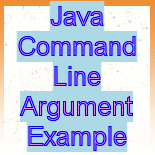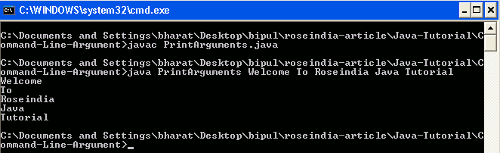# Java Command Line Argument Example

This section explains you how to take input through command line.### Java Command Line Argument Example

This section explains you how to take input through command line.## Java Command Line Argument Example

In this section we will read about how a Java programmer can allow the facility in the application that a user can provide the input using command line and the application produce the required output.

Command line argument is a way of providing input for the application. These inputs are given at the time of invoking application. Command line arguments are followed by a command for executing a class. Using command line argument a user can provide any number of arguments. The command line argument facilitate that the configuration information can be defined on launching of application. In an application command line argument is passed to the String array of its main method i.e. public static void main(String [] args) method.

Syntax :

```java className args1 args2 args3 .... argsN
```

In programming these command line arguments are termed as follows :

```args = args1;
args = args2;
....
....
args[N] = argsN;
```

e.g.

```java HelloWorld Welcome To Roseindia
```

Example

Here I am giving a simple example which will demonstrate you about how the command line argument can be provided to the Java application. In this example you will see that a simple Java program will be created into which the input for the application will be given from the command line. In this example we will create a Java program that will simply print all the arguments given from the command line at the console.

PrintArguments.java

```public class PrintArguments
{
public static void main(String args[])
{
for(String str : args)
{
System.out.println(str);
}
}
}
```

Output

First compile the above program and after successfully compiling the program execute the program and give the values for printing at console after the className followed by a white space as follows :

```javac PrintArguments.java
java PrintArguments Welcome To Roseindia Java Tutorial
```

Then the output will be as follows :